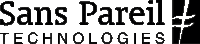BSON C++ API  2.5.1 uma::bson
uma::bson::ODMObject< Model >::MetaFieldImpl< DataType > Class Template Reference

Encapsulates a field in a model object. Fields are represented as a triplet of the field in the class, and its accessor and mutator methods. More...

`#include <ODMObject.h>`Inheritance diagram for uma::bson::ODMObject< Model >::MetaFieldImpl< DataType >:Collaboration diagram for uma::bson::ODMObject< Model >::MetaFieldImpl< DataType >:

## Public Types

typedef DataType &(Model::* Accessor )()
Member function pointer to the accessor method.

typedef void(Model::* Mutator )(const DataType &)
Member function pointer to the mutator method.

## Public Member Functions

MetaFieldImpl (const std::string &field, Accessor get, Mutator set)
Create a new field instance for the specified instance and methods.

ValuegetValue (Model *model)
Return the value for the field represented by this instance.

void setValue (Model *model, const Value &value)
Set the value for the field represented by this instance.Public Member Functions inherited from uma::bson::ODMObject< Model >::MetaField
MetaField (const std::string &field)
Create a new instance representing the field with the specified name.

virtual ~MetaField ()
Virtual dtor for sub-classing.

const std::string & getName () const
Return the name of the field encapsulated in this meta field instance.

## Detailed Description

### template<typename Model> template<typename DataType> class uma::bson::ODMObject< Model >::MetaFieldImpl< DataType >

Encapsulates a field in a model object. Fields are represented as a triplet of the field in the class, and its accessor and mutator methods.

Template Parameters
 Model The type of the class whose field is to be represented. This is implied by the template parameter for the wrapper ODMObject class. DataType The data type for the field. Must be a sub-class of uma::bson::Value.

## Member Typedef Documentation

template<typename Model >
template<typename DataType >
 typedef DataType&(Model::* uma::bson::ODMObject< Model >::MetaFieldImpl< DataType >::Accessor)()

Member function pointer to the accessor method.

template<typename Model >
template<typename DataType >
 typedef void(Model::* uma::bson::ODMObject< Model >::MetaFieldImpl< DataType >::Mutator)(const DataType &)

Member function pointer to the mutator method.

## Constructor & Destructor Documentation

template<typename Model >
template<typename DataType >
 uma::bson::ODMObject< Model >::MetaFieldImpl< DataType >::MetaFieldImpl ( const std::string & field, Accessor get, Mutator set )
inline

Create a new field instance for the specified instance and methods.

Parameters
 field The name of the field. get The accessor method for the field. set The mutator method for the field.

## Member Function Documentation

template<typename Model >
template<typename DataType >
 Value& uma::bson::ODMObject< Model >::MetaFieldImpl< DataType >::getValue ( Model * model )
inlinevirtual

Return the value for the field represented by this instance.

Parameters
 model The model object from which the value is to retrieved.
Returns
Value The value for the field.

Implements uma::bson::ODMObject< Model >::MetaField.

template<typename Model >
template<typename DataType >
 void uma::bson::ODMObject< Model >::MetaFieldImpl< DataType >::setValue ( Model * model, const Value & value )
inlinevirtual

Set the value for the field represented by this instance.

Parameters
 model The model object in which the value is to be stored. value The value for the field to store.

Implements uma::bson::ODMObject< Model >::MetaField.

The documentation for this class was generated from the following file:
• /Users/rakesh/projects/opensource/mongoviewer/bson/src/api/uma/bson/ODMObject.h